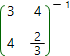# Inverse matrix online calculatorFor any non-singlar matrix (i.e. determinant doesn't equal to zero), exists inverse matrix, such as its product with initial matrix gives identity matrix:

A∙A−1 = A−1∙A = E

Our online calculator supports two different methods of matrix inverse calculation: by means of Gauss-Jordan method and by means of algebraic adjuncts compositions to the initial matrix.

To find inverse matrix by using Gauss-Jordan method, one needs to attach the identity matrix at the right of the initial matrix:

( A | E )

Then, by means of the elementary transformations, transform initial matrix to the identity one, applying the same transformations to the identity matrix written out at the right. Consequently, the initial matrix will be transformed to the identity one, and the identity matrix written out at the right - to the inverse one:

( A | E) → ( E | A−1 )

This method is easy, convenient and not so time-consuming.

To find the inverse matrix by means of algebraic adjunct method, one can use the following formula:where | A | - determinant of the matrix A,
Ai j - algebraic adjunct of the element ai j of the matrix A.

By the definition

Ai j = (-1) i+j Mi j

where Mi j - minor of the element ai j of the matrix A.

By the definition - minor of the element ai j of the matrix A is the determinant, received by removal i row, j column of the matrix A.

So, the algebraic adjunct method to find inverse matrix of the initial matrix of order n is very time-consuming, because one need to calculate not only the determinant of the initial matrix, but n2 determinants of order n-1 .

Inverse matrix calculatorA =